Structures 3 October/November 2015 Question Paper

Exam Year:2015

Free knec college past papers

This past paper applies for

STRUCTURES III
THE KENYA NATIONAL EXAMINATIONS COUNCIL
INSTRUCTIONS TO CANDIDATES

You should have Mathematical tables/Scientific calculator and drawing instruments for this examination.
This paper consists of EIGHT questions.
Answer any FIVE of the EIGHT questions in the spaces provided in this question paper.
All questions carry equal marks.
Maximum marks for each part of a question are as indicated.
Do NOT remove any pages from this booklet.
Candidates should answer the questions in English.

1. (a) State three advantages of the following connections:
(i) Bolted connections;
(ii) Welded connections.
(6 marks)
(b) Figure I shows a bolted connection required to transmit a tensile force of 250 KN.
Check the adequacy of the joint in terms of:
(i) Tensile stress in plates;
(ii) Tensile stress in angles;
(iii) hear stress in bolts;
(iv) Bearing stress in angles.
(14 marks)
Take the area of an 89 x 76 x 7.8 mm angle to be 12.35 cm2,
Permissible tensile stress = 155 N/mm2
Permissible shear stress = 80 N/mm2
Permissible bearing stress = 250 N/mm2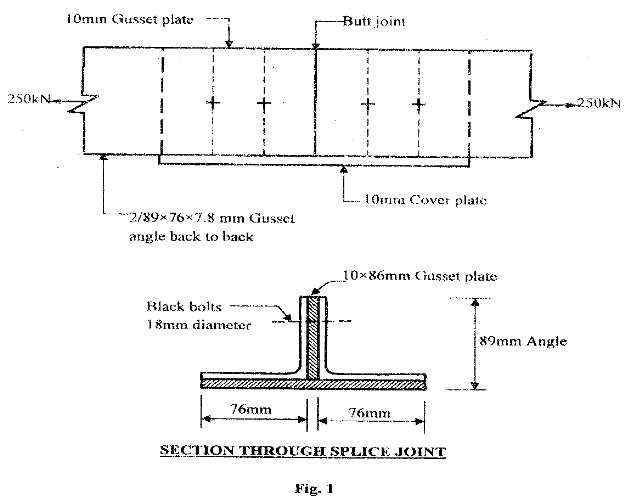2. (a) State four advantages of casing a steel section. (4 marks)

(b) A universal column used as an edge stanchion in a multi-storey building has an actual length of 3.6 m centre to centre of floor beam. The loading in the bean is as shown in figure 2. Design the stanchion as an encased column in Grade 43 steel, using the tables provided.
(16 marks)3. (a) Define the following terms as used to structure timber:
(i) Basic stress;
(ii) Green stress;

(b) A solid timber column of 200 mm x 150 mm and of strength of class 50 s is 4 m long
It is restrained in position and direction at both ends and is required to carry an axial load of 85 KN. Check the adequacy of the column.
– Table 9 ————— BS 5268
– Grade stress parallel to grain = 8.7 N/mm2
– Eminimum = 7.1 KN/mm2
– K3 = 1.25, Kg = 1.0, for medium duration.
(17 marks)
4. Using the moment distribution method, analyse the beam in figure 3 and sketch the bending moment diagram, indicating all critical values. (20 marks)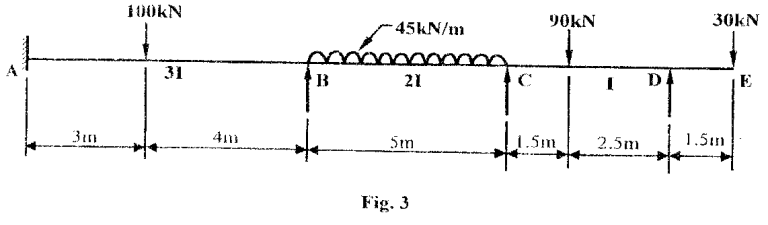5. Using the three moment theorem, analyse the bean shown in figure 4 and hence sketch the shear force and bending moment diagrams, indicating values at all critical points. (20 marks)6. Analyse the frame in figure 5 using moment of distribution method and then plot bending moment diagram, showing the values at all critical points. (20 marks)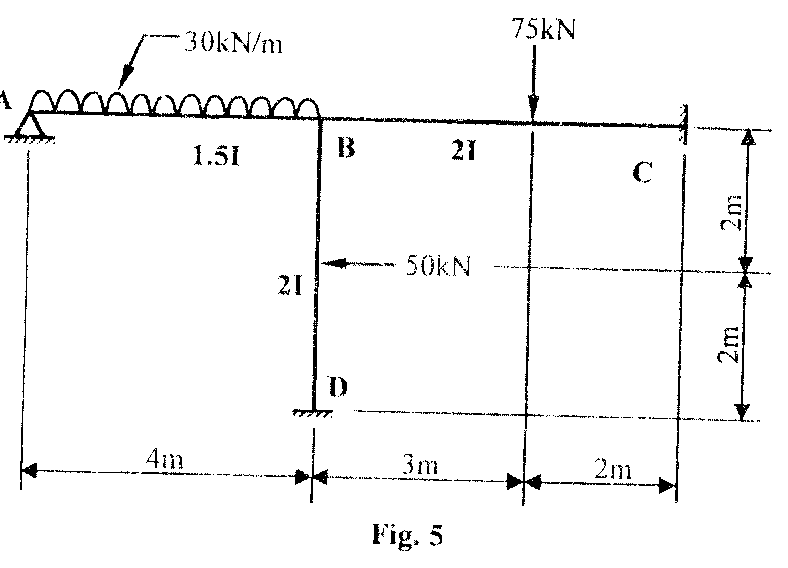7. (a) Figure 6 is a simply supported universal beam loaded as shown. Using the data provided below, check if a 533 x 165 x 73 kg/m UB will be satisfactory and hence check for shear and deflection.

Data
– Compression flanges fully restrained
Pq = 100N/mm2
E – 210 KN/mm2
(9 marks)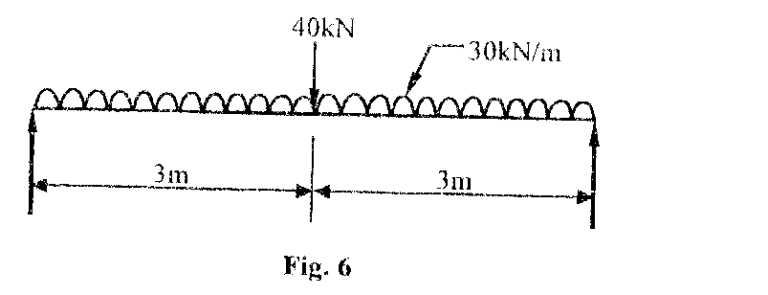-(b) (i) Sketch any two butt welds.

(ii) Design the connection in figure 7 shown using balanced weld design.
(11 marks)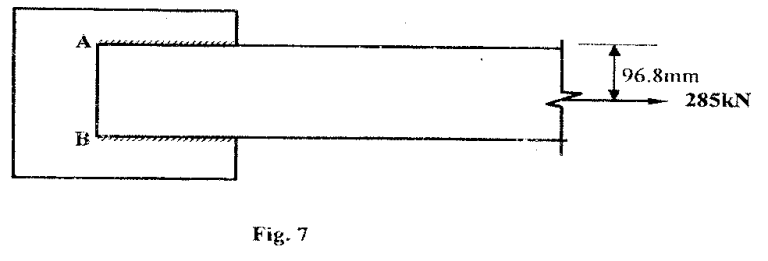8. (a) State five properties of structural timber as a construction material. (5 marks)
(b) A timber having a clear span of 6.0 m is suspended on 250 mm bearing at each end.
The beam carries a uniformly distributed load of 15 KN/m over the entire span.
Design the beam using the following information:
Permissible deflection = span/300
Permissible shear stress = l.2 Nmm2
Depth of section is twice the breadth
Young””s modulus of elasticity, E =8 KN/mm2
(15 marks)

More Related past papers

### Mathematics 3 And Surveying 3 October/November 2015 past paper